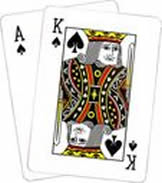Probability Practice Terms of Use    Contact Person: Donna RobertsDirections: Read carefully. Remember that a sample space, a tree diagram or a Venn diagram may be helpful.

1.
From a standard deck of cards, one card is drawn. What is the probability that the card is black and a jack?
Choose:
 1/2 1/13 1/26 1/52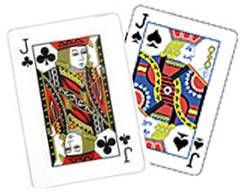2.
A die is rolled. What is the probability that the number rolled is greater than 2 and even?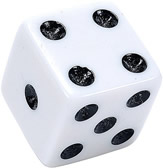Choose:
 1/2 1/3 2/3 5/6

3.
A set of polygons contains a square, a rectangle, a rhombus, and a trapezoid. If one polygon is chosen at random, what is the probability that the polygon has all sides equal in length and all right angles?
Hint: A square and a rhombus have all sides equal in length. A square and a rectangle have all right angles.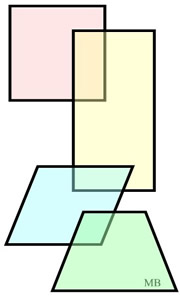Choose:
 1/4 1/3 1/2 3/4

4.
A piggy-bank contains 2 quarters, 3 dimes, 4 nickels, and 5 pennies. One coin is removed at random. What is the probability that the coin is a dime or a nickel?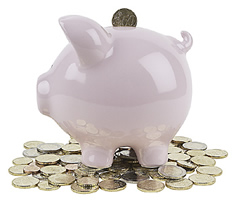Choose:
 3/14 4/14 7/14 1/7

5.
A bottle contains 3 red marbles, 5 blue marbles and 2 green marbles. If a single marble is drawn from the bottle at random, what is the probability that the marble is green or blue?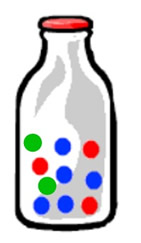Choose:
 1/2 1/5 3/5 7/10

6.
A standard deck of cards is shuffled and one card is drawn. Find the probability that the card is red or a jack.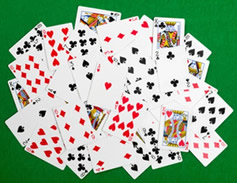Choose:
 7/52 1/13 7/13 1/26

7.
A bag contains 18 coins numbered from 1 to 18. Karen bets that the next coin drawn at random from the bag will be even. Cubby bets that the next coin drawn will be from the set of numbers {4, 5, 7, 10}. What is the probability that the next coin drawn will be even or from the set {4, 5, 7, 10}?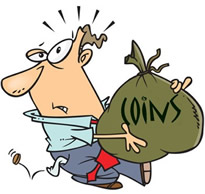Choose:
 4/18 9/18 11/18 13/18

8.
In a Math Honors class of 30 students, 16 are boys and 14 are girls. On the final exam, 7 boys and 8 girls received an "A" score. If a student is chosen at random from this class, what is the probability of choosing a girl or a student that received an "A" score?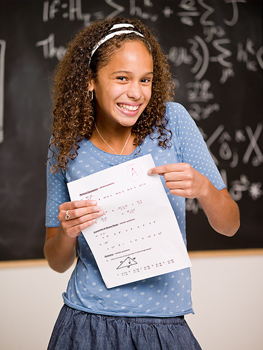Choose:
 21/30 29/30 15/30 24/30

9.
A spinner is divided into 8 congruent sectors. Set A = landing on a prime number.
Set B = landing on a multiple of 3.
When the spinner is spun one time, what is the P(AB)C ?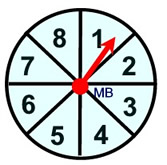Choose:
 1/8 5/8 6/8 7/8

10.
A card is chosen at random from a standard deck of 52 playing cards. There are 4 Kings and 4 Aces in a deck of standard playing cards. What is the probability that a card chosen at random from a deck of cards is a King or an Ace?
Choose:
 1/13 2/13 1/52 4/13